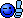# Maths help.

Discussion in 'General' started by Stickeh, 17 Apr 2004.

Joined:
20 Jul 2003
Posts:
3,574
89
eek i forgot to type anything in the thread - lol
here goes.
1. Simplify - (3xy³)²

2. Solve equation - 2x²-5x-4=0

3. P is inversely proportional to the square root of Q
If P=12 when Q=49 find an expression for P in the terms of Q.

if you can help please do!

Joined:
17 Apr 2003
Posts:
2,695
2
1 + 1 = 2

3. ### kenshinBanned

Joined:
22 Feb 2004
Posts:
351
0
2 / 2 = 1

Joined:
20 Jul 2003
Posts:
3,574
89
lol i know i forgot to to type anything auto hit ENTER instead of TAB lol.

5. ### UbermichHe did it!

Joined:
21 Jun 2002
Posts:
4,389
1
5 / 2 = 2
5 % 2 = 1

5 / 2 * 2 = 4
5 % 2 = 1
((5 / 2) * 2) + (5 % 2) = 5

correct me if I missed something.

Joined:
20 Jul 2003
Posts:
3,574
89
ok not funny any more... please i need the help.

7. ### ajackrox

Joined:
17 Apr 2003
Posts:
2,695
2
[EDIT] nah that was wrong...

8. ### kenshinBanned

Joined:
22 Feb 2004
Posts:
351
0
1) 9x² * y^6

3) P= A
Q^0.5​

12 = A
7​

P= 84Q

Can you check question two as i get roots which arent at gcse level.

Last edited: 17 Apr 2004
9. ### BjDWhat's a Dremel?

Joined:
10 Apr 2003
Posts:
935
0
2) dotn think it factorises cleanly at first glance, use the quadratic equation (x=-b+- squareroot(b^2-4ac) /2a) think thats how it goes3) P=-k * squareroot(Q)

sub P&Q to find k10. ### kenshinBanned

Joined:
22 Feb 2004
Posts:
351
0
-b ± squareroot (b²-4ac)
2a

Joined:
20 Jul 2003
Posts:
3,574
89
i dont know that equationim in year 11, prolly have learnt it just forgot

12. ### kenshinBanned

Joined:
22 Feb 2004
Posts:
351
0
you defo do the quadratic equation at gcse.

13. ### cpu121What's a Dremel?

Joined:
25 Nov 2003
Posts:
327
0
1) 9 * x^2 * y^6 (y^n)^a = y^n*a

2) -(-5) ± √ 5^2 - 4x2x-4
................2 x 2

5 ± √25 -(-32)
..........4

5 + √57 5 - √57
.......4....................................4

5 + 7.549834435 5 - 7.549834435
,,...........4...................................4

12.7549834435 -2.7.549834435
..........4..................................4

x = -0.6374586088 or 3.137458609 Round as required.

3) P = 84 * (1 / √q)

Hope that helps

Last edited: 17 Apr 2004
14. ### BjDWhat's a Dremel?

Joined:
10 Apr 2003
Posts:
935
0
ahh ah, i see i made a small error in my other postit should be...
P=k * (1/root(Q))

so sub for P&Q to find k15. ### Der Me!\$terWhat's a Dremel?

Joined:
14 Sep 2002
Posts:
535
0
2) Don't know if you mean -2x²-5x-4=0 or 2x²-5x-4=0

a = (-)2
b = -5
c = -4

plug'em in et voila (-2x²-5x-4=0):

x1 = 11/2
x2 = 8

or if it should be 2x²-5x-4=0

x1 = 21/2
x2 = 8

I learnt that formula in 10th grade igcse as well...

16. ### SanchosWhat's a Dremel?

Joined:
13 Mar 2004
Posts:
25
0
-b ± squareroot (b²-4ac)
2a

The method is actually given to you in the gcse paper, with how to find the area of sphere ect, therefore u dont need to memorise that method, just how to use it to solve quadratics.

17. ### kenshinBanned

Joined:
22 Feb 2004
Posts:
351
there is also completing the square but i wont get into that